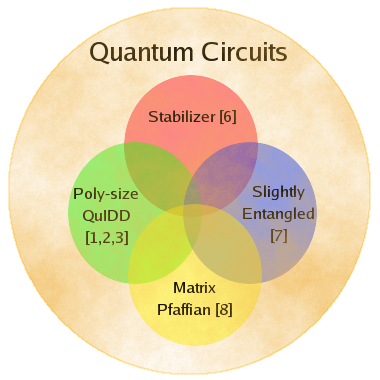# Relation to Other Techniques

In addition to QuIDDs, other simulation algorithms have been proposed to enable efficient simulation of quantum circuits [8, 9, 10]. Each of these simulation algorithms can efficiently simulate its own unique class of quantum circuits. QuIDDs too enable efficient simulation of yet another unique class of quantum circuits, and an important subset of this class is formally defined in , which includes instances of Grover's quantum search algorithm. In general, QuIDDs enable efficient simulation when the relevant matrices contain blocks of repeated values. A high-level depiction of the unique class of quantum circuits that can be simulated efficiently by QuIDDs is shown along with the other unique classes that can be simulated efficiently by the other techniques.As indicated by the figure, there is overlap in the classes of circuits that each algorithm can simulate efficiently. In addition, there are subsets of each class which can be simulated efficiently by the corresponding algorithm alone. As a result, QuIDDPro should not be viewed as a competing quantum circuit simulator, but rather as a computational interface to one of several known algorithms that simulates a unique class of quantum circuits efficiently. This interface can be used as a computational back-end engine to any existing or future quantum circuit simulator.